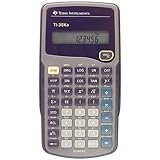» » Barcode: 0999992087369Tag# Texas Instruments TI-30Xa Scientific Calculator( Audio Instrument Product )Rank: 100%Texas Instruments TI-30Xa Scientific Calculator

Specifications

• Product color is Grey

• Product weight is 0.3 lbs.
Adds, subtracts, multiplies, and divides fractions entered in traditional numerator/denominator format Performs trigonometric functions, logarithms, roots, powers, reciprocals, and factorials. Polar/rectangular conversions Battery powered Adds, subtracts, multiplies, and divides fractions entered in traditional numerator/denominator format Performs trigonometric functions, logarithms, roots, powers, reciprocals, and factorials Polar/rectangular conversions 1-variable statistics include results for mean and standard deviation Battery powered; slide case included Basic, battery-operated scientific calculator with one-line display and general math and science functionality. Key Features: One-line, 10-digit display Fraction features One-variable statistics Conversions Basic scientific and trigonometric functions Ideal For: General math Pre-Algebra Algebra 1 and 2 General science Once you get to a certain level of math classes, a regular old calculator just isn't good enough anymore--you need a high-functioning calculator that can perform algebraic, trigonometric, and statistical problems in addition to general mathematical tasks. The Texas Instruments TI-30XA scientific calculator can do this for you. It is extremely handy when it comes to figuring tricky fractions. It can add, subtract, multiply, and divide fractions when entered in a traditional numerator/denominator format. This calculator can also do a variety of conversions, from fractions to decimals, degrees to radians to grads, DMS to decimal to degrees, and polar to rectangular. The display screen holds up to 10 digits.For high school or college students tackling advanced math, this is a great tool (and a thoughtful back-to-school gift), and with more professors allowing the use of calculators during classes and exams, it's a necessary one. Easy to use and easy to slip into a backpack, the TI-30XA will soon become indispensable for both the serious and the striving math student. --Dana Van Nest

References
^ Texas Instruments TI-30Xa Scientific Calculator, Texas Instruments. Amazon. (revised Feb 2015)

 Page 1 of 1 1
 Page 1 of 1 1

## Texas Instruments TI-30Xa Scientific Calculator (2022-07-02)

### Account

Social:
Pages:  ..   ..
Items:  ..

Help:  ..   ..
Category:  ..   ..
Media:  ..   ..
Posts:  ..   ..   ..

### Statistics

Page:  ..
Summary:  ..
1 Tags
10/10 Page Rank
4 Page Refs
6s Time
32 Sources